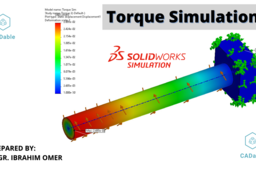# SOLIDWORKS Torque Simulation | Shaft Analysis | 3 Simulation Studies | Torque Simulation| CADableIn this tutorial, we'll learn basic static torque simulation in SOLIDWORKS 2020. First of all, we'll model a solid shaft with a rectangular plate and then we'll do simulation. Here, I have used Axis, Split Line features which will be used when we apply load (torque) on the shaft. Don't forget to add simulation add-in before making static simulation study. The simulation contains the following steps:

3) Apply Boundary Conditions (Free Body Diagram)
4) Meshing the Model
5) Run the Analysis (Solve)
6) View the Results

After completing the first simulation, I have created two more simulations by changing the position of the applied torque. Remember I have used same:

a) Material
b) Geometry
c) Boundary Conditions
d) Torque (100 N.m)
e) Mesh Density

The only thing I have change in simulation two and three, is the position of applied torque. And here you will see the difference. This difference is not so big but it is there. I have also shown true scale